# 基于BPNN-GARCH模型的互联网货币基金收益率预测Forecasting the Rate of Return of Internet Monetary Fund Based on BPNN-GARCH Model

DOI: 10.12677/AAM.2017.69138, PDF, HTML, XML, 下载: 969  浏览: 5,950

Abstract: On the basis of the traditional time series method, a nonlinear BP neural network model is intro-duced, and a combined model of generalized autoregressive conditional heteroskedasticity model and BP neural network model is established to predict the yield of the Internet monetary fund. The prediction accuracy of three models was compared with four indexes of MAE, MSE, ME and DA. Based on the empirical analysis, the BPNN-GARCH combined model has higher accuracy for the forecast of the Internet monetary fund yield.

1. 引言

2013年6月，在利率市场化的背景下，余额宝正式上线，这是第三方支付平台支付宝与天弘基金合作推出的兼具理财和消费支付功能的互联网理财产品，也是我国第一支互联网货币基金。此后半年内其迅猛发展，成为国内首支规模破千亿的基金。截止2017年3月31日，余额宝资产规模达到11,396.38亿元，比上期增长40.99%。相比于传统的货币基金，余额宝取消了最低门槛限制，简化了开户流程，増加了随时消费支付，收益即时分配到账等功能。不仅实现了技术创新和渠道创新，并且提高了对碎片化资金的利用效率。在强大的示范效应下，基金公司、互联网平台纷纷推出各种“宝宝”类理财产品加入混战。然而目前对于互联网货币基金收益率波动性及收益率预测的研究还较为缺乏，金融资产的风险一般表现为收益的不确定性，在对互联网货币基金收益率的预测的基础上，可以对互联网货币基金的风险特征进行更多的分析，促进基金的稳定发展，提高投资者的风险意识，保护投资者的利益。因此，对于互联网货币基金收益率的预测具有重要的现实意义。

1986年，Rumelhart和Meclland提出了误差反向传播算法(BP算法)。随着研究的逐渐深入，多种人工神经网络模型被提出并得以深入研究，但BP神经网络及它的改进形式约占现有神经网络模型的80%~90%。BP神经网络具有结构简单，可操作性强、较好的自学习和自适应能力、能模拟任意的非线性输入和输出关系等优点。目前神经网络已被广泛用于模式识别、智能控制、预测、图像识别、函数拟合、系统仿真等领域。对于经济预测方面，Matsuba (1991)率先将神经网络应用到估值预测中  。其后，Liu，Yao (2001)运用基于进化算法的前馈神经网络对香港恒生指数进行了预测，取得了良好的结果  。而刘澄等(2009)利用基于遗传算法的BP神经网络模型拟合股指波动趋势，并选取上证指数为样本进行实证分析  。Wang Guo (2011)建立以小波变换为基础的BP神经网络模型来预测股票价格波动，对上证综合指数的月度数据展开分析，经过实证对比发现，经小波变换法优化处理的BP神经网络模型输出相对较优  。

2. 模型介绍

2.1. BP神经网络模型

BP神经网络是一种按误差逆传播算法训练的多层前馈网络，是目前应用最广泛的神经网络模型之一。它的学习规则是使用最速下降法，通过反向传播来调整网络的权值和阈值，使网络的误差平方和最小。BP神经网络由输入层、隐藏层、输出层三层构成  。

$y=f\left(\underset{i=1}{\overset{n}{\sum }}{\omega }_{i}{x}_{i}-\theta \right),$ (1)

$E=\frac{1}{2}\underset{k=1}{\overset{l}{\sum }}{\left({d}_{k}-{o}_{k}\right)}^{2}.$ (2)

$\left\{\begin{array}{l}\Delta {\omega }_{jk}=\eta {\delta }_{k}^{0}{y}_{j}=\eta \left({d}_{k}-{o}_{k}\right){o}_{k}\left(1-{o}_{k}\right){y}_{j}\\ \Delta {v}_{ij}=\eta {\delta }_{j}^{y}{x}_{i}=\eta \left(\underset{k=1}{\overset{l}{\sum }}{\delta }_{k}^{0}{\omega }_{jk}\right){y}_{j}\left(1-{y}_{j}\right){x}_{i}\end{array}$ (3)

$\eta$ 为学习速率， ${\delta }_{k}^{0},{\delta }_{j}^{y}$ 为误差信号。

2.2. GARCH模型

$\left\{\begin{array}{l}{y}_{i}=\varphi x+{u}_{t},{u}_{t}~N\left(0,{\sigma }_{t}^{2}\right)\\ {\sigma }_{t}^{2}={\alpha }_{0}+\underset{i=1}{\overset{p}{\sum }}{\alpha }_{i}{u}_{t-1}^{2}\end{array}$ (4)

$\left\{\begin{array}{l}{y}_{i}=\varphi x+{u}_{t},{u}_{t}~N\left(0,{\sigma }_{t}^{2}\right)\\ {\sigma }_{t}^{2}={\alpha }_{0}+\underset{i=1}{\overset{p}{\sum }}{\alpha }_{i}{u}_{t-1}^{2}+\underset{j=1}{\overset{q}{\sum }}{\alpha }_{j}{\sigma }_{t-1}^{2}\end{array}$ (5)

2.3. 组合模型

$\stackrel{^}{y}=\underset{i=1}{\overset{m}{\sum }}{w}_{i}{\stackrel{^}{y}}_{i},\text{\hspace{0.17em}}\text{\hspace{0.17em}}\underset{i=1}{\overset{m}{\sum }}{w}_{i}=1$ (6)

$\stackrel{^}{y}={w}_{1}{\stackrel{^}{y}}_{\text{GARCH}}+{w}_{2}{\stackrel{^}{y}}_{\text{BPNN}},\text{\hspace{0.17em}}\text{\hspace{0.17em}}\text{\hspace{0.17em}}{w}_{1}+{w}_{2}=1$ (7)

$\stackrel{^}{y}={w}_{1}{\stackrel{^}{y}}_{\text{BPNN}}+{w}_{2}{\stackrel{^}{y}}_{\text{GARCH}}$ (8)

3. 实证分析

3.1. 数据选取和评价标准

$\text{MAE}=\frac{\underset{i=1}{\overset{n}{\sum }}|{\stackrel{^}{y}}_{i}-{y}_{i}|}{n}$ (9)

$\text{MSE}=\frac{\underset{i=1}{\overset{n}{\sum }}{\left({\stackrel{^}{y}}_{i}-{y}_{i}\right)}^{2}}{n}$ (10)

$\text{ME}=\frac{\underset{i=1}{\overset{n}{\sum }}\left({\stackrel{^}{y}}_{i}-{y}_{i}\right)}{n}$ (11)

$\text{DA}=\frac{\underset{i=1}{\overset{n}{\sum }}{d}_{i}}{n},\text{\hspace{0.17em}}\text{\hspace{0.17em}}{d}_{i}=\left\{\begin{array}{l}1,\left({y}_{i}-{y}_{i-1}\right)\left({\stackrel{^}{y}}_{i}-{\stackrel{^}{y}}_{i-1}\right)>0\\ 0,其它\end{array}$ (12)

3.2. 建立GARCH模型

3.2.1. 描述性检验

3.2.2. GARCH模型的建立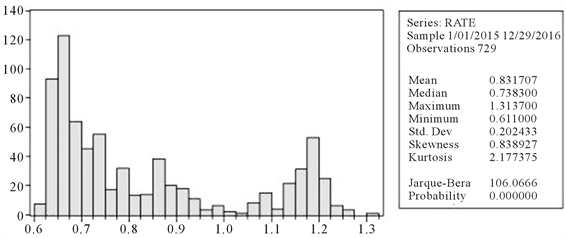Figure 1. Sample data descriptive chart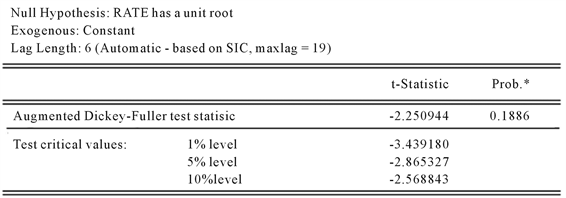Figure 2. Yield sequence stability test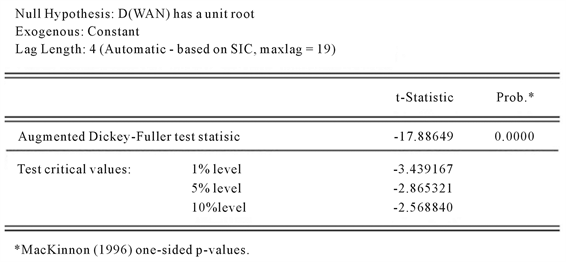Figure 3. First order differential stability test

${y}_{t}=-0.000274-0.56{y}_{t-1}-0.339{y}_{t-2}-0.218{y}_{t-3}-0.076{y}_{t-4}+{\epsilon }_{t},{u}_{t}~N\left(0,{\sigma }_{t}^{2}\right)$

${\sigma }_{t}^{2}=0.00087+0.085{u}_{t-1}^{2}-0.248{\sigma }_{t-1}^{2}$

3.3. 建立BP神经网络模型

3.3.1. BP神经网络各参数的确定

3.3.2. BP神经网络模型的预测

3.4. 建立BPNN-GARCH组合模型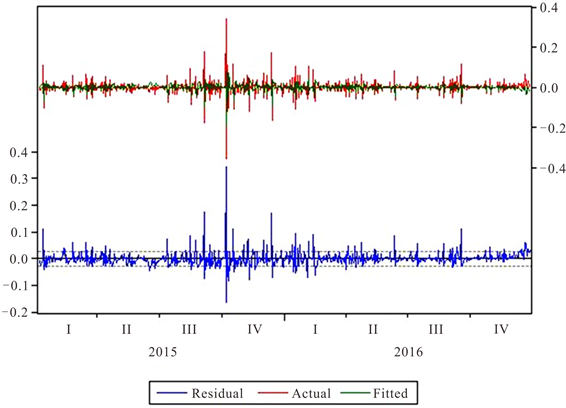Figure 4. GARCH (1,1) model fitting renderingsFigure 5. GARCH (1,1) model forecast graphFigure 6. BP neural network model forecast graph

$\stackrel{^}{y}=0.56{\stackrel{^}{y}}_{\text{BPNN}}+0.44{\stackrel{^}{y}}_{\text{GARCH}\left(1,1\right)}$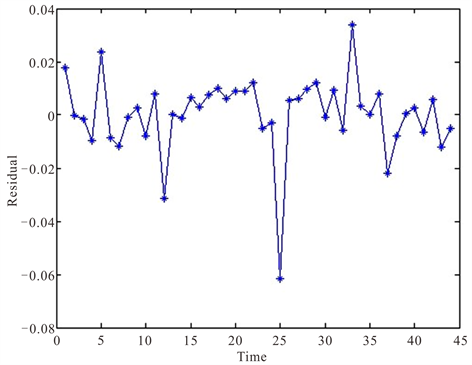Figure 7. BP neural network model residual graph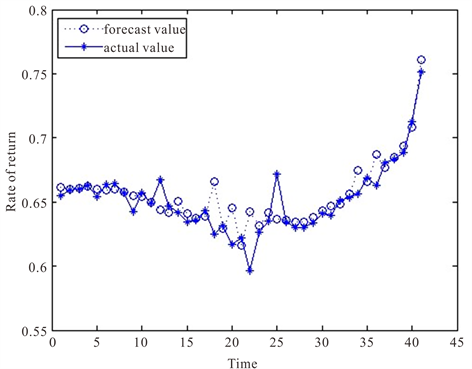Figure 8. Combined model forecast graph

3.5. 结果分析

4. 结束语

BPNN-GARCH模型在各项检验指标中有三项指标位均优于GARCH模型和BP神经网络模型。说明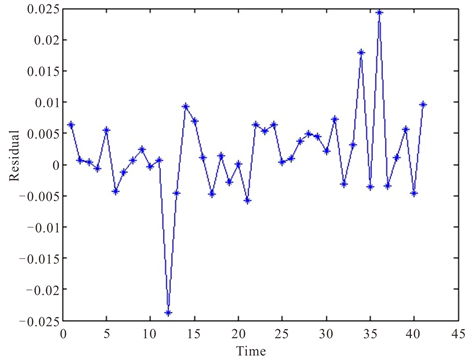Figure 9. Combined model residual graphTable 1. Comparison of prediction effect of each model

BPNN-GARCH组合模型在互联网货币基金余额宝的收益率预测中更具有优势。利用BPNN-GARCH组合模型对互联网货币基金进行收益率预测具有更高的准确率。但组合模型仍存在极端值预测不准确的问题，如何改进模型使其更加容易捕捉互联网货币基金收益率的极端值，仍需进一步的研究。

  周正清. 欧美货币市场基金监管改革对我国T+0货币市场基金发展的启示[J]. 南方金融, 2014(4): 58-61.  赵舒怡, 李敬湘. 互联网货币市场基金的发展优势及潜在风险[J]. 时代金融, 2015, 12(610): 120-121.  Tang, Z., de Almeida, C. and Fishwick, P.A. (1991) Time Series Forecasting Using Neural Networks vs. Box-Jenkins Method-ology. Simulation, 57, 303-310. https://doi.org/10.1177/003754979105700508  Liu, Y. and Yao, X. (2001) Evolving Neural Networks for Hang Seng Stock Index Forecast. Proceedings of the 2001 Congress on Evolutionary Computation, 1, 256-260.  刘澄, 王燕, 孙彬. GA-BP算法在金融股指预测中的应用研究[J]. 技术经济与管理研究, 2009(6): 16-18.  Wang, J.Z., Wang, J.J., Zhang, Z.-G. and Guo, S.-P. (2011) Forecasting Stock Indices with Back Propagation Neural Network. Expert Systems with Applications, 38, 14346-14355. https://doi.org/10.1016/j.eswa.2011.04.222  Bates, J.M. and Garanger, C.W.J. (1969) The Combination of Forecasts. Operational Research Quarterly, 20, 451-468. https://doi.org/10.1057/jors.1969.103  Sallehuddin, R. and Shamsuddin, S.M. (2008) Hybridization Model of Linear and Nonlinear Time Series Data for Forecasting. Second Asia International Conference on Modelling Simulation, 1 May 2008, 597-602.  张东, 安玉娥, 傅娟. 一种改进的基于指数平滑神经网络模型的时间序列预测方法[J]. 数学的实践与认识, 2013, 43(5): 20-28.  韩力群. 人工神经网络理论、设计及应用[M]. 北京: 化学工业出版社, 2007.  Domian, D.L. and Reichenstein, W. (1997) Performance and Persistence in Money Market Fund Returns. Financial Services Review, 6, 169-183. https://doi.org/10.1016/S1057-0810(97)90011-1